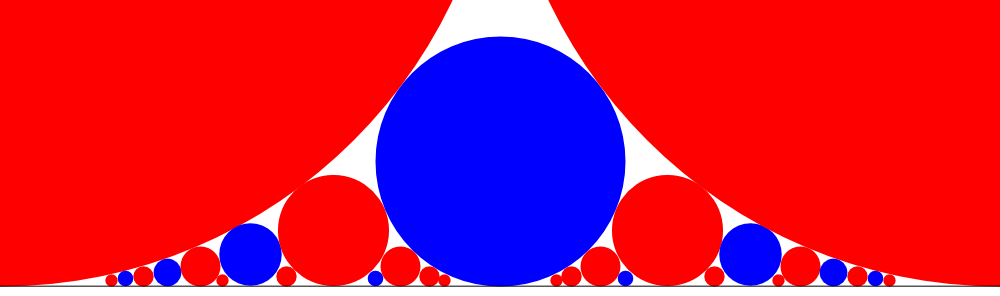## The Ford Circles Game

What are the Ford circles? A picture is worth a thousand words, so here is a picture.We draw a circle for any rational number p/q between 0 and 1 inclusive. We assume that p/q is the representation of the number in the lowest terms. Then the center of the circle is located at (p/q,1/q) and the radius is 1/q. The number inside a circle is q.

Here’s the game. We start with any circle on the picture, except for the two largest circles corresponding to integers 0 and 1. In one move we can switch to a larger circle that touches our circle. The person who ends up at the two largest circles corresponding to integers 0 or 1 loses. Equivalently, the person who ends in the central circle marked “2” wins.

There are other ways to describe moves in this game in terms of rational numbers corresponding to circles, that is, the x-coordinates of their centers. Two circles corresponding to numbers p/q and r/s touch each other iff one of the following equivalent statements is true:

• The cross-determinant of two numbers p/q and r/s that is defined as |ps-qr| equals to 1;
• p/q and r/s are neighbors in some Farey sequence;
• One of the numbers is the parent of the other in the Stern-Brocot tree.

Let me explain the last bullet. Given two rational numbers in their lowest terms a/b and c/d, we generate their mediant as: (a+c)/(b+d). We call the two numbers a/b and c/d the parents of the mediant (a+c)/(b+d). The Stern-Brocot tree starts with two parents 0/1 and 1/1. Then their mediant is inserted between them to create a row: 0/1, 1/2, and 1/1. Then all possible mediants of two consecutive numbers are inserted in a given row to get a new row. The process repeats ad infinitum. The famous theorem states that any rational number between 0 and 1 will appear in the process.

What I like about this game is a simple and beautiful description of P-positions (These are the positions you want to end your move at in order to win.) P-positions are numbers with even denominators in their lowest terms.In the picture above P-positions are blue, while other positions are red. All circles touched by blue are red. And if we look at the larger neighbors of every red circle, one of them is blue and one is red.

Let’s prove that the numbers with even denominators satisfy the conditions for P-positions. First, two circles corresponding to numbers with even denominators can’t touch each other. Indeed, the cross-determinant of two such fractions is divisible by 2. Second, each red circle has to touch one blue and one red circle with larger radii. Indeed, the circles with larger radii touching a given circle are exactly the parents of the circle. If the mediant has an odd denominator, then one of the parents must have an even denominator and the other an odd denominator.

Share: